# Power Inductor Basic Course - Chapter 1-2

Contents：Chapter 1: What is a Power Inductor?
 1.1 Overview of power inductors 1.2 Basic characteristics of power inductors 1.3 Types of power inductors 1.4 Power inductor selection issues
1.2 Basic characteristics of power inductors

Now what parameters should you pay attention to when selecting a power inductor? The power inductor catalogs of each manufacturer list the following principal specifications. Figure 1-2 shows an example of a specification table.

 ◆Inductance ◆Rated current... DC superposition rated current Isat Temperature increase rated current Itemp ◆DC resistance Rdc ◆Operating temperature range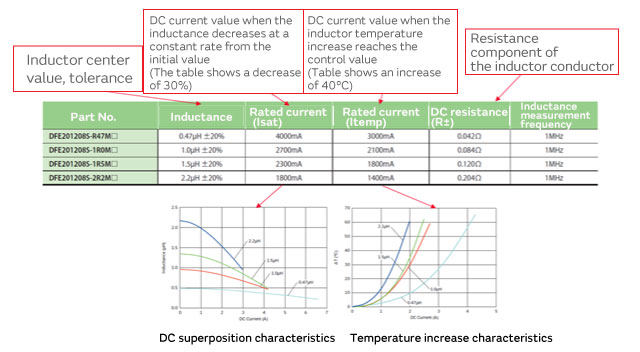Figure 1-2 Power inductor characteristic table

However, we don’t know the right specification for which power inductor to choose with only this information. For example, questions such as whether a high or low inductance is better, or what rated current is required, must be considered to appropriately choose an inductor according to the operating conditions of the DC-DC converter. In this chapter, we will cover how to read power inductor specifications.

＜Inductance＞

The inductance value is an extremely important parameter which affects the ripple current and load response characteristics. As shown in Figure 1-3, an exponential current flows through a power inductor used in a DC-DC converter. Generally speaking, it is considered to be a good idea to set the ripple current ⊿IL to about 30% of the load current Iout. Therefore, once the DC-DC converter conditions are decided, you can calculate the proper power inductor inductance with the following equation.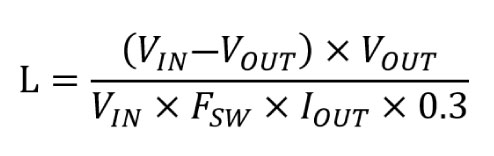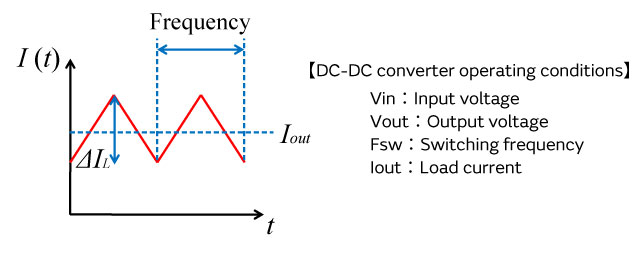Figure 1-3 Current waveform flowing through a power inductor

However, most DC-DC converters list the proper inductance value as a reference. Therefore, you can also follow the manufacturer reference to select the power inductor without calculating the formula above.

＜Rated current＞
The rated current stipulates the current value at which the quality can no longer be guaranteed once the DC current exceeds it. The rated current of a power inductor stipulates a value for the DC superposition rated current (Saturation) and a value for the temperature increase rated current (Temperature). The meaning of each value is important, so in many cases they are listed as separate specifications.

ⅰ）DC superposition rated current Isat
One characteristic of an inductor is the DC superposition characteristic. To obtain a high inductance, ferrite and other magnetic materials are used in the core (magnetic core) of the inductor. When a current flows through the inductor, the phenomenon called the magnetic saturation of the magnetic substance occurs, and the inductance decreases. This characteristic is called “DC superposition.” The DC superposition rated current stipulates the current value when this inductance decreases at a constant rate with regard to the initial characteristic that the current is not superimposed on.

ⅱ）Temperature increase rated current Itemp
This is the rated current requirement which uses component heat generation as an index and use beyond this range will lead to component and set damage. Typically, it is defined as the current value where the temperature increase is ⊿40°C.

Now let’s look at how to determine these rated currents when used as a power inductor. As shown in Figure 1-3, the maximum current which flows through the inductor is Iout + ⊿IL/2. When this current value exceeds Isat, the decrease in inductance becomes quite large; the shape of the exponential current becomes abnormal as shown by the red line in Figure 1-4, and the ripple current increases. Because the ripple current causes the output voltage to fluctuate, it induces abnormal system behavior on the load side when the ripple current increases. Therefore, you must choose a power inductor with an Isat that is greater than the maximum current.
At the same time, with regard to the temperature increase rated current, the inductor is not immediately damaged even if the rated value is exceeded. Accordingly, you should select an Itemp value which is greater than Iout as a general rule.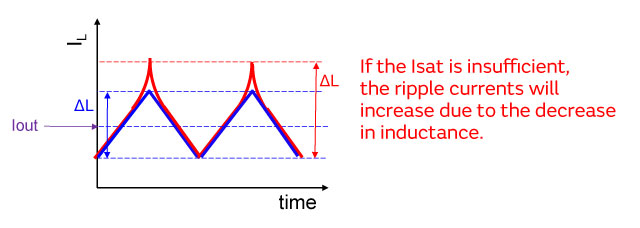Figure 1-4 Ripple current fluctuations due to the DC superposition

＜DC resistance Rdc＞
The DC resistance is the resistance value when the direct current flows. Because power loss occurs through heat generation due to this resistance value, a lower DC resistance can reduce loss. However, reducing the Rdc involves a trade-off between the DC superposition characteristic and size miniaturization. Therefore, it is probably better to select an inductor with a smaller Rdc from among those inductors which satisfy the necessary characteristics such as inductance and the rated currents discussed above.

＜Operating temperature range＞
The operating temperature range stipulates the allowable range of ambient temperature when using an inductor. The effect of temperature can change even based on the circuit operating environment, so please select an inductor based on the expected practical use environment.

Now let’s take a look at an example of selecting a power inductor in an actual DC-DC converter. This example is based on the step-down DC-DC converter shown in Figure 1-5. It is expected to operate under the following conditions:

 [Operating conditions] Vin：3.6V Vout：1.8V FSW：2.0MHz Iout：1.5A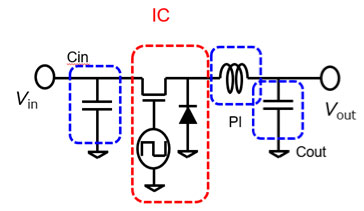Figure 1-5 Step-down DC-DC converter example

With the following equation, we see that the magnitude of the proper inductance is about 1.0 μH.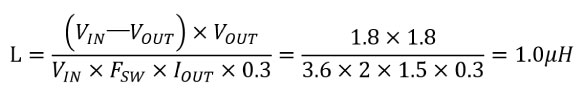Moreover, because Iout = 1.5 A and ⊿IL is 0.45 A at about 30% of Iout, the maximum current is as follows:
Iout+⊿IL/2＝1.725A
Therefore, based on this result, we need an inductor with an Itemp of 1.5 A or more and an Isat of 1.8 A or more.

By using Murata’s design support software called the “SimSurfing / DC-DC Converter Design Support Tool,” you can search for the ideal item based on the required specification. For example, when the required values for inductance, size, Itemp, and Isat are entered from among the search items shown within the red frame in Figure 1-6, the items which match the conditions are listed within the blue frame.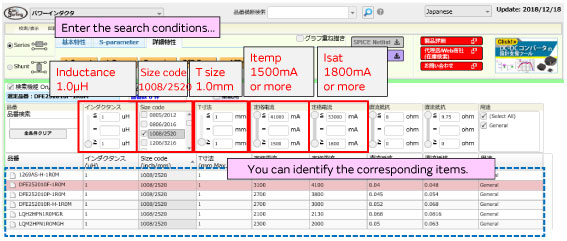Figure 1-6 Search conditions in the “SimSurfing” design support software

You can also compare basic characteristics such as the DC superposition characteristic by selecting items.

In Figure 1-7, the following three items are selected to compare the superposition characteristic:
DFE252010F-1R0M
LQH2HPN1R0MGR
LQM2HPN1R0MGH
Based on these results, for this example, we can see that the DFE252012P-1R0M satisfies the required conditions and possesses low Rdc and high Isat characteristics.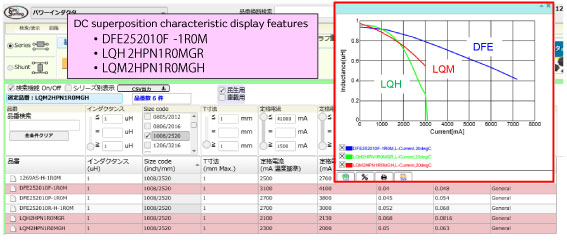Figure 1-7 Comparison of basic characteristics with the “SimSurfing” design support software

Contents：Chapter 1: What is a Power Inductor?
 1.1 Overview of power inductors 1.2 Basic characteristics of power inductors 1.3 Types of power inductors 1.4 Power inductor selection issues